Courses

# H2 and Br2 react to give HBr by the following steps: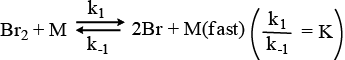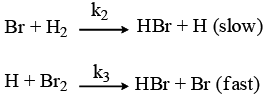The probable rate law for the above sequence is a)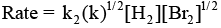b)Rate = k2 [H2][Br2]c)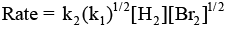d)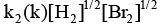Correct answer is option 'A'. Can you explain this answer? Related Test: Test: Chemical Kinetics - 2

## Chemistry QuestionRam Kumar Feb 04, 2019
This is little bit easy, dear.. First, you should write the rate equation for slowest step, then for [Br], go to 1st step and as it is in equilibrium, so rate of forward reaction = rate of backward reaction. After this step, you'll be able to get the answer, which is option A.

This discussion on H2 and Br2 react to give HBr by the following steps:The probable rate law for the above sequence isa)b)Rate = k2 [H2][Br2]c)d)Correct answer is option 'A'. Can you explain this answer? is done on EduRev Study Group by Chemistry Students. The Questions and Answers of H2 and Br2 react to give HBr by the following steps:The probable rate law for the above sequence isa)b)Rate = k2 [H2][Br2]c)d)Correct answer is option 'A'. Can you explain this answer? are solved by group of students and teacher of Chemistry, which is also the largest student community of Chemistry. If the answer is not available please wait for a while and a community member will probably answer this soon. You can study other questions, MCQs, videos and tests for Chemistry on EduRev and even discuss your questions like H2 and Br2 react to give HBr by the following steps:The probable rate law for the above sequence isa)b)Rate = k2 [H2][Br2]c)d)Correct answer is option 'A'. Can you explain this answer? over here on EduRev! Apart from being the largest Chemistry community, EduRev has the largest solved Question bank for Chemistry.

### Related Content

This discussion on H2 and Br2 react to give HBr by the following steps:The probable rate law for the above sequence isa)b)Rate = k2 [H2][Br2]c)d)Correct answer is option 'A'. Can you explain this answer? is done on EduRev Study Group by Chemistry Students. The Questions and Answers of H2 and Br2 react to give HBr by the following steps:The probable rate law for the above sequence isa)b)Rate = k2 [H2][Br2]c)d)Correct answer is option 'A'. Can you explain this answer? are solved by group of students and teacher of Chemistry, which is also the largest student community of Chemistry. If the answer is not available please wait for a while and a community member will probably answer this soon. You can study other questions, MCQs, videos and tests for Chemistry on EduRev and even discuss your questions like H2 and Br2 react to give HBr by the following steps:The probable rate law for the above sequence isa)b)Rate = k2 [H2][Br2]c)d)Correct answer is option 'A'. Can you explain this answer? over here on EduRev! Apart from being the largest Chemistry community, EduRev has the largest solved Question bank for Chemistry.